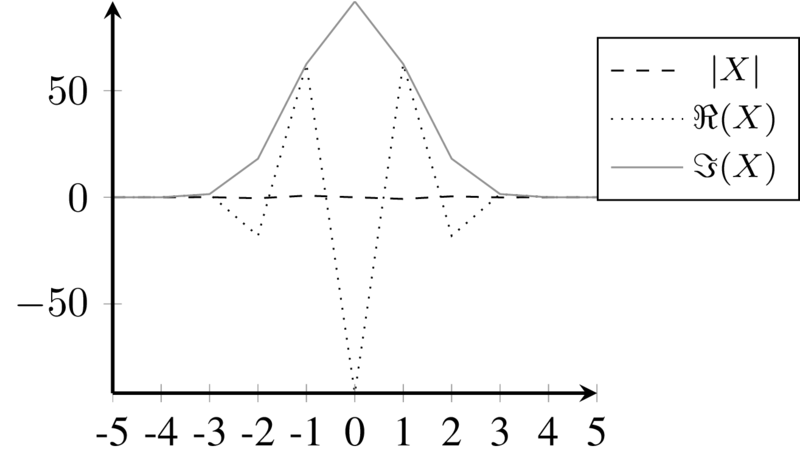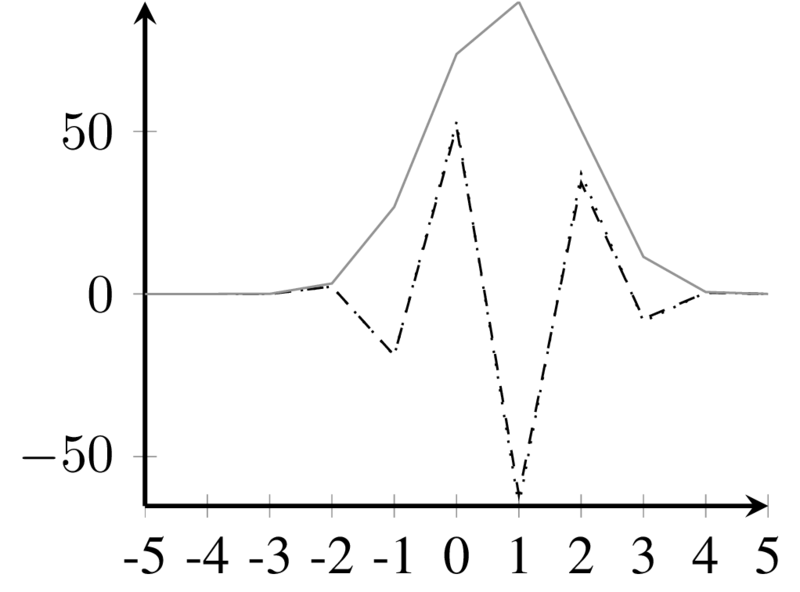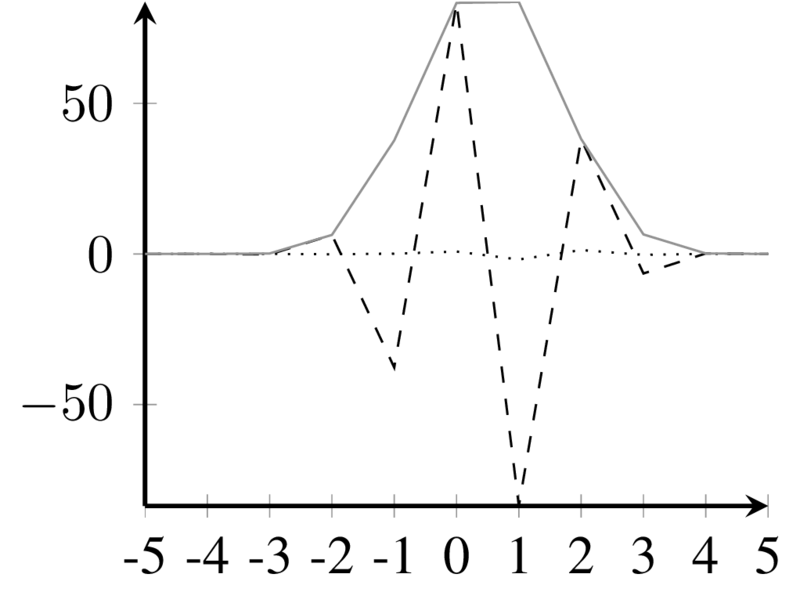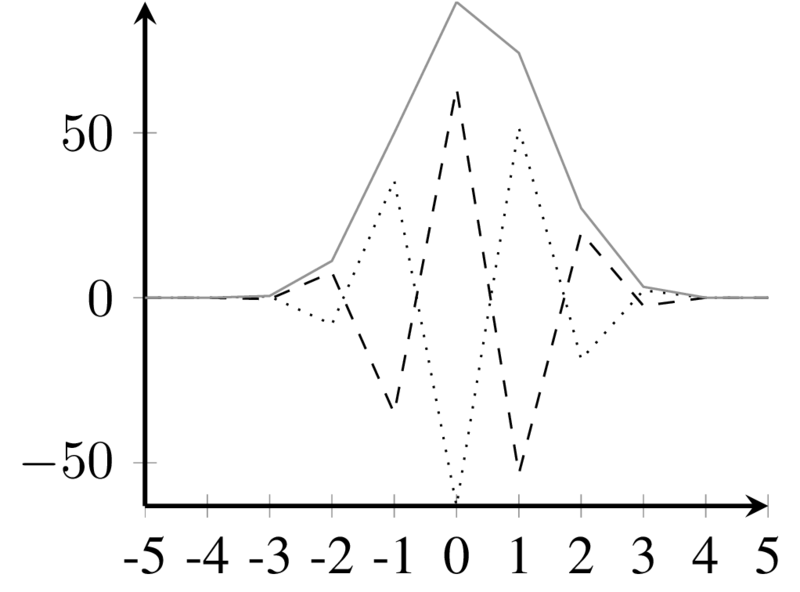# Additive & Spectral: IFFT Synthesis

The calculation of single sinusoidal components in the time domain can be very inefficient for a large number of partials. IFFT synthesis can be used to compose spectra in the frequency domain.Main lobe kernel for $\varphi = 0$Main lobe kernel for $\varphi = \pi/2$Main lobe kernel for $\varphi = \pi/4$Main lobe kernel for $\varphi =c3 \pi/4$